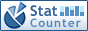Home PC Games Linux Windows Database Network Programming Server Mobile
 Home \ Programming \ Java rewrite the hashcode method - Source code compiled by the installation program under Linux (Linux) - Python common data type summary (Programming) - Redis logging system (Database) - Linux resource restriction level summary (Linux) - CentOS 6.4 dial-up Raiders (Linux) - RedHat Linux 7 build yum source server (Server) - Zabbix monitoring Oracle Database use Orabbix plug (Enhanced Edition) (Database) - To configure linux transparent firewall (Linux) - Linux System Getting Started Learning: Disable HTTP forwarding wget in (Linux) - Using RAID in Linux: Create a RAID 5 (Linux) - Bash added to the Vi mode indicator (Linux) - Linux user status query, and to unlock locked user (Linux) - Using nmcli commands to manage network in RedHat / CentOS 7.x (Linux) - Magical Virtualbox under Ubuntu (Linux) - PostgreSQL log classification and management (Database) - C ++ handling text input (Programming) - Neo4j map data processing tab (Database) - CentOS6 5 Configure SSH password Free (Linux) - Linux CPU Monitoring Index (Linux) - Quick Install software RAID on Linux (Linux) Java rewrite the hashcode method Add Date : 2018-11-21 Override hashcode 1. a non-zero constant value, such as 17, stored in the int variables result in; (Each domain refers to the equals method considered) 2. For each object, a key field f: 3, boolean type, calculate (f 0: 1?); 4. byte, char, short type, calculate (int); 5. long type, calculate (int) (f ^ (f >>> 32)); 6. float type, calculated Float.floatToIntBits (afloat); 7. double type, the calculation Double.doubleToLongBits (adouble) to give a long, and then perform [2.3]; 8. The object reference, call the object .hashCode () method; 9. domain object array, where each element of the recursive call its hashCode method. 10. Basic array of domains, where each element of the calculation according to the type of returns 2 11. Save the above calculated hash code to the int variable c, and then perform result = 37 * result + c; 12. Return result. example:     / ****     *     *     * Java eight basic data types     * /     private int A_int;     private short A_short;     private char A_char;     private byte A_byte;     private double A_double;     private float A_float;     private boolean A_boolean;     private long A_long;     private Demo demo; // Object     private int [] intArray; // array field     private Demo [] demos; // array of objects domain      @Override     public int hashCode () {         int result = 17;         result = 31 * result + A_int;         result = 31 * result + (int) A_short;         result = 31 * result + (int) A_char;         result = 31 * result + (int) A_byte;         result = 31 * result + (int) (A_boolean 0:? 1);         result = 31 * result + (int) (A_long ^ (A_long >>> 32));         result = 31 * result + Float.floatToRawIntBits (A_float);         long tolong = Double.doubleToLongBits (A_double);         result = 31 * result + (int) (tolong ^ (tolong >>> 32));                  result = 31 * result + demo.hashCode (); // object         result = 31 * result + intArrayHashcode (intArray); // array of fields, each element of which call its hashCode method.         result = 31 * result + DemoArrayHashcode (demos); // array of objects domain, a recursive call its hashCode method                  return result;     }     private int intArrayHashcode (int [] intArray) {         int result = 17;         for (int i = 0; i < intArray.length; i ++) {// primitive array field, where each element of the calculation             result = 31 * result + intArray [i];         }         return result;     }     private int DemoArrayHashcode (Demo [] demos) {         int result = 17;         for (int i = 0; i < demos.length; i ++) {             result = 31 * result + demos [i] .hashCode (); // array of objects domain, a recursive call its hashCode method;         }         return result;     } When the rewrite equals (), always to rewrite hashCode () According equals method of a class (before rewriting), two distinct instances of possible logically equal, but, according to Object.hashCode method, they are only two objects. Thus, the violation of the "Equal objects must have equal hash codes." ps: 31 is a magical number because any number n * 31 can be optimized for the JVM (n < < 5) Operating efficiency -n, shift and subtraction multiplication than high operational efficiency and more to the left and now many virtual machine which has to do related optimization, and 31 occupied 5bits! More:
 CopyRight 2002-2022 newfreesoft.com, All Rights Reserved.